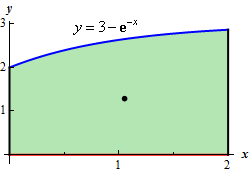Paul's Online Notes
Home / Calculus II / Applications of Integrals / Center of Mass
Show Mobile Notice Show All Notes Hide All Notes
Mobile Notice
You appear to be on a device with a "narrow" screen width (i.e. you are probably on a mobile phone). Due to the nature of the mathematics on this site it is best views in landscape mode. If your device is not in landscape mode many of the equations will run off the side of your device (should be able to scroll to see them) and some of the menu items will be cut off due to the narrow screen width.

### Section 2-3 : Center Of Mass

2. Find the center of mass for the region bounded by $$y = 3 - {{\bf{e}}^{ - x}}$$, the $$x$$-axis, $$x = 2$$ and the $$y$$-axis.

Show All Steps Hide All Steps

Start Solution

Let’s start out with a quick sketch of the region, with the center of mass indicated by the dot (the coordinates of this dot are of course to be determined in the final step…..).We’ll also need the area of this region so let’s find that first.

$A = \int_{0}^{2}{{3 - {{\bf{e}}^{ - x}}\,dx}} = \left. {\left( {3x + {{\bf{e}}^{ - x}}} \right)} \right|_0^2 = 5 + {{\bf{e}}^{ - 2}}$ Show Step 2

Next, we need to compute the two moments. We didn’t include the density in the computations below because it will only cancel out in the final step.

\begin{align*}{M_x} & = \int_{0}^{2}{{\frac{1}{2}{{\left( {3 - {{\bf{e}}^{ - x}}} \right)}^2}\,dx}} = \int_{0}^{2}{{\frac{1}{2}\left( {9 - 6{{\bf{e}}^{ - x}} + {{\bf{e}}^{ - 2x}}} \right)\,dx}} = \left. {\frac{1}{2}\left( {9x + 6{{\bf{e}}^{ - x}} - \frac{1}{2}{{\bf{e}}^{ - 2x}}} \right)} \right|_0^2 = \frac{{25}}{4} + 3{{\bf{e}}^{ - 2}} - \frac{1}{4}{{\bf{e}}^{ - 4}}\\ {M_y} & = \int_{0}^{2}{{x\left( {3 - {{\bf{e}}^{ - x}}} \right)\,dx}} = \int_{0}^{2}{{3x - x\,{{\bf{e}}^{ - x}}\,dx}} = \left. {\left( {\frac{3}{2}{x^2} + x{{\bf{e}}^{ - x}} + {{\bf{e}}^{ - x}}} \right)} \right|_0^2 = 5 + 3{{\bf{e}}^{ - 2}}\end{align*}

For the second term in the $${M_y}$$ integration we used the following integration by parts.

\begin{align*} & \int{{x\,{{\bf{e}}^{ - x}}\,dx}}\hspace{0.25in}\hspace{0.25in}u = x\,\,\,\,\,\,du = dx\hspace{0.25in}\hspace{0.25in}dv = {{\bf{e}}^{ - x}}dx\,\,\,\,\,v = - {{\bf{e}}^{ - x}}\\ & \int{{x\,{{\bf{e}}^{ - x}}\,dx}} = - x{{\bf{e}}^{ - x}} + \int{{{{\bf{e}}^{ - x}}\,dx}} = - x{{\bf{e}}^{ - x}} - {{\bf{e}}^{ - x}} = - \left( {x{{\bf{e}}^{ - x}} + {{\bf{e}}^{ - x}}} \right)\end{align*}

The minus sign here canceled with the minus sign that was in front of the term in the full integral.

Make sure you don’t forget integration by parts! It is a fairly common integration technique for these kinds of problems.

Show Step 3

Finally, the coordinates of the center of mass is,

$\overline{x} = \frac{{{M_y}}}{M} = \frac{{\rho \left( {5 + 3{{\bf{e}}^{ - 2}}} \right)}}{{\rho \left( {5 + {{\bf{e}}^{ - 2}}} \right)}} = 1.05271\hspace{0.25in}\hspace{0.25in}\overline{y} = \frac{{{M_x}}}{M} = \frac{{\rho \left( {\frac{{25}}{4} + 3{{\bf{e}}^{ - 2}} - \frac{1}{4}{{\bf{e}}^{ - 4}}} \right)}}{{\rho \left( {5 + {{\bf{e}}^{ - 2}}} \right)}} = 1.29523$

The center of mass is then : $$\require{bbox} \bbox[2pt,border:1px solid black]{{\left( {1.05271,\,\,1.29523} \right)}}$$.